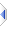# remainder

 Definition: atom a3 = remainder(atom a1, atom a2) Description: Compute the remainder after dividing a1 by a2. The result will have the same sign as a1, and the magnitude of the result will be less than the magnitude of a2. Comments: This function may be applied to an atom or sq_rmdr() to all elements of a sequence. The rules for sequence operations apply. Example 1: ```a = remainder(9, 4) -- a is 1 ``` Example 2: ```s = sq_rmdr({81, -3.5, -9, 5.5}, {8, -1.7, 2, -4}) -- s is {1, -0.1, -1, 1.5} ``` Example 3: ```s = sq_rmdr({17, 12, 34}, 16) -- s is {1, 12, 2} ``` Example 4: ```s = sq_rmdr(16, {2, 3, 5}) -- s is {0, 1, 1} ``` Implementation: via :%opRmdr in builtins\VM\pRmdr.e (an autoinclude) See Also: floor, mod